How Cheenta works to ensure student success?
Explore the Back-Story

# ISI B.Math 2005 Subjective Paper| Problems & Solutions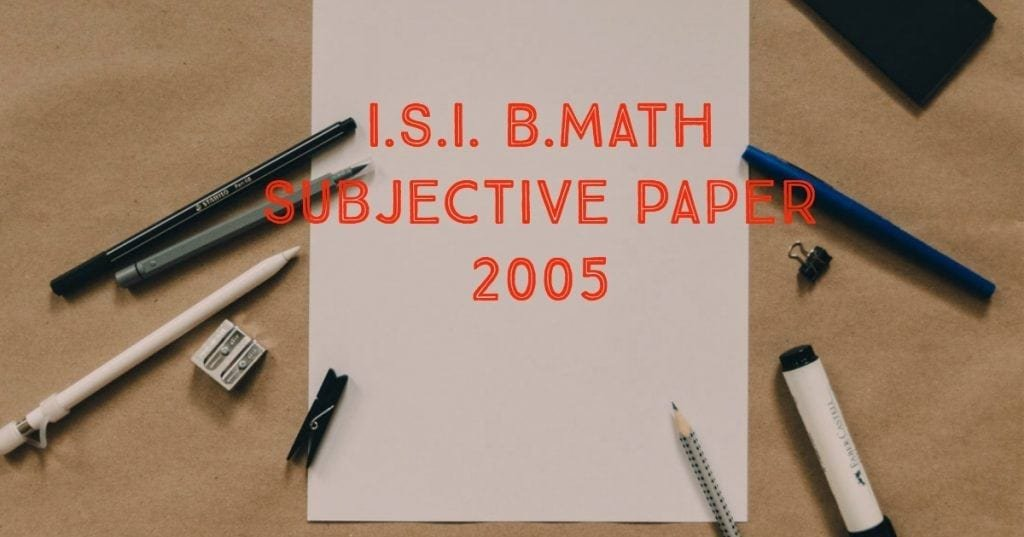Here, you will find all the questions of ISI Entrance Paper 2005 from Indian Statistical Institute's B.Math Entrance. You will also get the solutions soon of all the previous year problems.

Problem 1 :

For any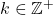, prove that:-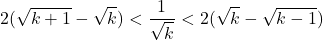Also compute integral part of.

Problem 2 :

Let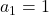and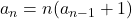for all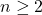. Define :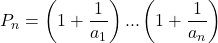Compute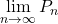Problem 3 :

Let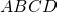be a quadrilateral such that the sum of a pair of opposite sides equals the sum of other pair of opposite sides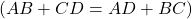. Prove that the circles inscribed in trianglesandare tangent to each other.

Problem 4 :

For a set S we denote its cardinality by |S|. Let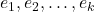be non-negative integers. Let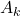(respectively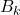) be the set of all k-tuples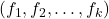of integers such that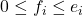for all i and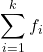is even (respectively odd). Show that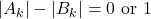.

Problem 5 :

Find the point in the closed unit disc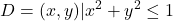at which the function f(x,y)=x+y attains its maximum .

Problem 6 :

Let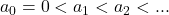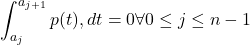Show that, for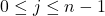, the polynomial p(t) has exactly one root in the intervalProblem 7 :

Let M be a point in the triangle ABC such that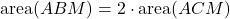Show that the locus of all such points is a straight line.

Problem 8 :

In how many ways can one fill an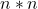matrix with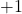and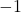so that the product of the entries in each row and each column equals?

Here, you will find all the questions of ISI Entrance Paper 2005 from Indian Statistical Institute's B.Math Entrance. You will also get the solutions soon of all the previous year problems.

Problem 1 :

For any, prove that:-Also compute integral part of.

Problem 2 :

Letandfor all. Define :ComputeProblem 3 :

Letbe a quadrilateral such that the sum of a pair of opposite sides equals the sum of other pair of opposite sides. Prove that the circles inscribed in trianglesandare tangent to each other.

Problem 4 :

For a set S we denote its cardinality by |S|. Letbe non-negative integers. Let(respectively) be the set of all k-tuplesof integers such thatfor all i andis even (respectively odd). Show that.

Problem 5 :

Find the point in the closed unit discat which the function f(x,y)=x+y attains its maximum .

Problem 6 :

LetShow that, for, the polynomial p(t) has exactly one root in the intervalProblem 7 :

Let M be a point in the triangle ABC such thatShow that the locus of all such points is a straight line.

Problem 8 :

In how many ways can one fill anmatrix withandso that the product of the entries in each row and each column equals?

This site uses Akismet to reduce spam. Learn how your comment data is processed.

### Knowledge Partner